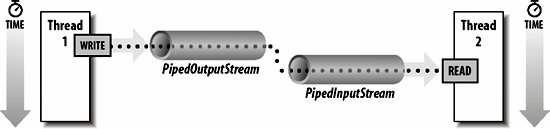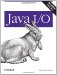# Communicating Between Threads Using Piped Streams

The java.io.PipedInputStream class and java.io.PipedOutputStream classes provide a convenient means to move data from one thread to another. Output from one thread becomes input for the other thread, as shown in Figure 9-1.

Figure 9-1. Data moving between threads using piped streams```public class PipedInputStream extends InputStream
public class PipedOutputStream extends OutputStream
```

The PipedInputStream class has two constructors:

```public PipedInputStream( )
public PipedInputStream(PipedOutputStream source) throws IOException
```

The no-argument constructor creates a piped input stream that is not yet connected to a piped output stream. The second constructor creates a piped input stream that's connected to the piped output stream source.

The PipedOutputStream class also has two constructors:

```public PipedOutputStream(PipedInputStream sink) throws IOException
public PipedOutputStream( )
```

The no-argument constructor creates a piped output stream that is not yet connected to a piped input stream. The second constructor creates a piped output stream that's connected to the piped input stream sink.

Piped streams are normally created in pairs. The piped output stream becomes the underlying source for the piped input stream. For example:

```PipedOutputStream pout = new PipedOutputStream( );
PipedInputStream pin = new PipedInputStream(pout);
```

This simple example is a little deceptive because these lines of code will normally be in different methods and perhaps even different classes. Some mechanism must be established to pass a reference to the PipedOutputStream into the thread that handles the PipedInputStream. Or you can create them in the same thread, then pass a reference to the connected stream into a separate thread. Alternately, you can reverse the order:

```PipedInputStream pin = new PipedInputStream( );
PipedOutputStream pout = new PipedOutputStream(pin);
```

Or you can create them both unconnected, then use one or the other's connect( ) method to link them:

```PipedInputStream pin = new PipedInputStream( );
PipedOutputStream pout = new PipedOutputStream( );
pin.connect(pout);
```

Otherwise, these classes just have the usual read( ), write( ), flush( ), close( ), and available( ) public methods like all stream classes.

PipedInputStream also has four protected fields and one protected method that are used to implement the piping:

```protected static final int PIPE_SIZE
protected byte[] buffer
protected int in
protected int out
protected void receive(int b) throws IOException
```

PIPE_SIZE is a named constant for the size of the buffer. The buffer is the byte array where the data is stored, and it's initialized to be an array of length PIPE_SIZE. When a client class invokes a write( ) method in the piped output stream class, the write( ) method invokes the receive( ) method in the connected piped input stream to place the data in the byte array buffer. Data is always written at the position in the buffer given by the field in and read from the position in the buffer given by the field out.

There are two possible blocks here. The first occurs if the writing thread tries to write data while the reading thread's input buffer is full. When this occurs, the output stream enters an infinite loop in which it repeatedly waits for one second until some thread reads some data out of the buffer and frees up space. If this is likely to be a problem for your application, subclass PipedInputStream and make the buffer larger. The second possible block is when the reading thread tries to read and no data is present in the buffer. In this case, the input stream enters an infinite loop in which it repeatedly waits for one second until some thread writes some data into the buffer.

Although piped input streams contain an internal buffer, they do not support marking and resetting. The circular nature of the buffer would make this excessively complicated. You can always chain the piped input stream to a buffered input stream and read from that if you need marking and resetting.

The following program is a simple and somewhat artificial example that generates Fibonacci numbers in one thread and writes them onto a piped output stream while another thread reads the numbers from a corresponding piped input stream and prints them on System.out. This program uses three classes: FibonacciProducer and FibonacciConsumer, which are subclasses of Thread, and FibonacciDriver, which manages the other two classes. Example 9-3 shows the FibonacciProducer class, a subclass of Thread. This class does not directly use a piped output stream. It just writes data onto the output stream that it's given in the constructor.

Example 9-3. The FibonacciProducer class

 ```import java.io.*; public class FibonacciProducer extends Thread { private DataOutputStream theOutput; private int howMany; public FibonacciProducer(OutputStream out, int howMany) { theOutput = new DataOutputStream(out); this.howMany = howMany; } public void run( ) { try { int f1 = 1; int f2 = 1; theOutput.writeInt(f1); theOutput.writeInt(f2); // Now calculate the rest. for (int i = 2; i < howMany; i++) { int temp = f2; f2 = f2 + f1; f1 = temp; if (f2 < 0) { // overflow break; } theOutput.writeInt(f2); } } catch (IOException ex) { System.err.println(ex); } } } ```

Example 9-4 is the FibonacciConsumer class. It could just as well have been called the IntegerConsumer class since it doesn't know anything about Fibonacci numbers. Its run( ) method merely reads integers from its input stream until the stream is exhausted. At this point, the other end of the pipe closes and an IOException is thrown. The only way to tell the difference between this normal termination and a real exception is to check the exception message.

Example 9-4. The FibonacciConsumer classm

 ```import java.io.*; public class FibonacciConsumer extends Thread { private DataInputStream theInput; public FibonacciConsumer(InputStream in) { theInput = new DataInputStream(in); } public void run( ) { try { while (true) { System.out.println(theInput.readInt( )); } } catch (IOException ex) { if (ex.getMessage( ).equals("Pipe broken")) { // normal termination return; } System.err.println(ex); } } } ```

Example 9-5 is the FibonacciDriver class. It creates a piped output stream and a piped input stream and uses those to construct FibonacciProducer and FibonacciConsumer objects. These streams are a channel of communication between the two threads. As data is written by the FibonacciProducer thread, it becomes available for the FibonacciConsumer tHRead to read. Both the FibonacciProducer and the FibonacciConsumer are run with normal priority so that when the FibonacciProducer blocks or is preempted, the FibonacciConsumer runs and vice versa.

Example 9-5. The FibonacciDriver class

 ```import java.io.*; public class FibonacciConsumer extends Thread { private DataInputStream theInput; public FibonacciConsumer(InputStream in) { theInput = new DataInputStream(in); } public void run( ) { try { while (true) { System.out.println(theInput.readInt( )); } } catch (IOException ex) { if (ex.getMessage( ).equals("Pipe broken") || ex.getMessage( ).equals("Write end dead")) { // normal termination return; } ex.printStackTrace( ); } } } ```

You may be wondering how the piped streams differ from the stream copiers presented earlier in the book. The first difference is that the piped stream moves data from an output stream to an input stream. The stream copier always moves data in the opposite direction, from an input stream to an output stream. The second difference is that the stream copier actively moves the data by calling the read( ) and write( ) methods of the underlying streams. A piped output stream merely makes the data available to the input stream. It is still necessary for some other object to invoke the piped input stream's read( ) method to read the data. If no other object reads from the piped input stream, after about one kilobyte of data has been written onto the piped output stream, the writing thread blocks while it waits for the piped input stream's buffer to empty.Java I/O
ISBN: 0596527500
EAN: 2147483647
Year: 2004
Pages: 244